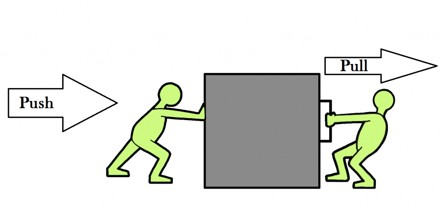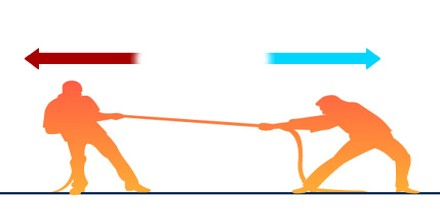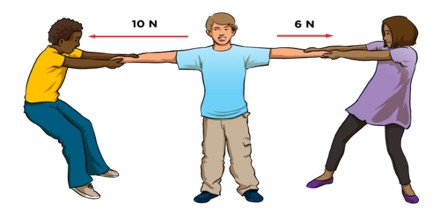Force

Definition

Force is a dynamic influence that changes a body from a state of rest to one of motion or changes its rate of motion. The magnitude of the force is equal to the product of the mass of the body and its acceleration. It can also be described intuitively as a push or a pull. A force has both magnitude and direction, making it a vector quantity. It is measured in the SI unit of newtons and represented by the symbol F.Force is proportional to acceleration. In calculus terms, force is the derivative of momentum with respect to time. The standard unit of force is the newton. One newton is equivalent to one kilogram-meter per second squared (kg · m/s 2 or kg · m · s -2). Alternatively, the dyne is sometimes used to express force; one dyne is equal to one gram-centimeter per second squared (g · cm/s 2 or g · cm · s -2). To convert from newtons to dynes, mulitiply by 100,000 (10 5). Conversely, multiply by 0.00001 (10 -5).

The Newton

Force is a quantity that is measured using the standard metric unit known as the Newton. A Newton is abbreviated by an “N.” To say “10.0 N” means 10.0 Newton of force. One Newton is the amount of force required to give a 1-kg mass an acceleration of 1 m/s/s. Thus, the following unit equivalency can be stated: 1 Newton = 1 kg • m/s2Foundamental Forces

There are four fundamental forces that govern the interactions of physical systems. Scientists continue to pursue a unified theory of these forces.

Gravitation is the force that acts between masses. All particles experience the force of gravity. While the graviton has been proposed as the particle mediating gravity, it has not yet been observed.

The electromagnetic force acts between electrical charges and the mediating particle is the photon.

The strong nuclear force holds the nucleus of the atom together, mediated by gluons acting on quarks, antiquarks, and the gluons themselves.

The weak nuclear force is mediated by exchanging W and Z bosons, and is seen in beta decay of neutrons in the nucleus. At very high temperatures, the weak force and the electromagnetic force are indistinguishable.Applications of Force

Practical applications of force are everywhere from rockets to cars to just about anything. Force can be in many forms to get some form of work done, be it lifting, pushing, electrical current, to atomic and sub atomic levels.

For example to take a step forward, we need friction against the floor, our muscles have to shift our centre of gravity forward so that we are essentially starting to fall face first slightly. This act places a force on the ground in the backwards direction which coupled with friction and our body mass being accelerated by gravity towards the Earth, resists our pushing backwards on it.  Our muscles then have to apply a variety of forces to our other leg (rotational and upwards) to lift our foot off of the ground. These forces are equally transferred and resisted through your other leg to the ground.

With modern insights into quantum mechanics and technology that can accelerate particles close to the speed of light, particle physics has devised a Standard Model to describe forces between particles smaller than atoms. The Standard Model predicts that exchanged particles called gauge bosons are the fundamental means by which forces are emitted and absorbed.

Vector Quantity

A force is a vector quantity. As learned in an earlier unit, a vector quantity is a quantity that has both magnitude and direction. A force is a vector that has a direction; it is common to represent forces using diagrams in which a force is represented by an arrow. Such vector diagrams were introduced in an earlier unit and are used throughout the study of physics. The size of the arrow is reflective of the magnitude of the force and the direction of the arrow reveals the direction that the force is acting.